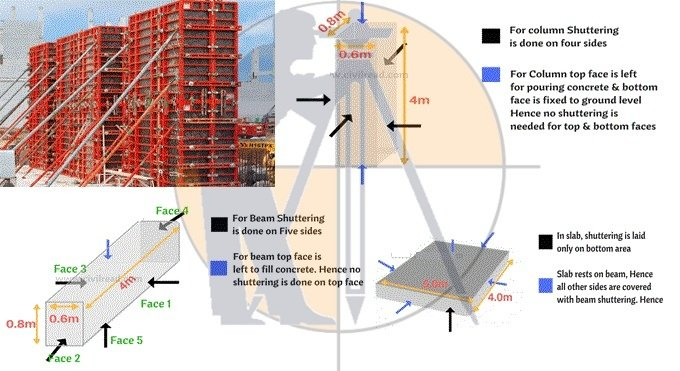Breaking News
Home / Civil Engineering / How To Calculate The Shuttering Area For Beam, Column And Slab

# How To Calculate The Shuttering Area For Beam, Column And Slab

### How To Calculate The Shuttering Area For Beam, Column And Slab

Shuttering stands for a temporary structure formed to retain wet concrete that is poured in it to produce roofs and concreting walls in building structures.

It supports and retains the liquid concrete until it achieves the required strength and shape. When the structure becomes sufficiently strong to bear its load, this temporary structure is detached.How to measure the shuttering area:

The calculation of shuttering is done with sq.m in the rate analysis of shuttering. To measure the area of shuttering, it is required to know the process for working out peripheral length (perimeter) of an any shape.

### Civil Engineering Tips | Points to Remember For Civil Site Engineer

Peripheral length (Perimeter):

Perimeter belongs to the span around a two dimensional shape.
Given below, some vital formulas for measuring the shuttering area:
Perimeter of Square : 4S (S denotes Length of each side)
Perimeter of Rectangle : 2[L+B] (L & B denotes length & breadth)
Perimeter of Circle : 2πr (r denotes radius of circle)
Area of Rectangle = Length x Breadth
Area of Square = Side x Side

It should be kept in mind that each member in a structure ranging from slab or beam or column contains six sides (faces). The measurement for shuttering area is done with two methods. One is done with formula and other is by measuring the specific areas of faces.

The following formula is used :-

Shuttering area = Peripheral length (Perimeter) x Depth
Computation of shuttering area of a column
Suppose, the column shuttering is performed on four sides.
Top face of the column is discarded for pouring concrete & the bottom face is secured to ground level. Here, the calculation should not done for top & bottom faces.

Toward column, shuttering is carried out for four sides and the other two sides.
The side of column remains in rectangular shape having side length as l and breadth as b.

Peripheral length of rectangular = l+b+l+b = 2l + 2b
Shuttering area = peripheral length (perimeter) x depth
Peripheral length = 2×0.8 + 2×0.6 = 1.6 + 1.2 = 2.8 sq.m
So, total area of shuttering for a column = 2.8 x 4 = 11.2 sq.m
When the shape of column is circular, then the following formula is used to work out the shuttering area.

2πr x Depth
Measurement of shuttering area for a beam
The shuttering is provided on five sides for a beam.
In beam, the top face is departed to fill concrete. Therefore, no shuttering is arranged on top face.

#### HOW TO CALCULATE STEEL QUANTITY FOR SLAB WITH BAR BENDING SCHEDULE

The calculation is done by determining the separate area of each faces as follow :-

Face 1 : Area of rectangle = L x B = 0.8 x 4 = 3.2
Face 2 : Area of rectangle = L x B = 0.6 x 4 = 2.4
Face 3 : Area of rectangle = L x B = 0.8 x 4 = 3.2
Face 4 : Area of rectangle = L x B = 0.8 x 0.6 = 0.48
Face 5 : Area of rectangle = L x B = 0.8 x 0.6 = 0.48

Total Area of Shuttering = 3.2 + 2.4 + 3.2+ 0.48 +0.48 = 9.76.Sqm

Measurement of Shuttering Area of a Slab:-

Slab is supported on beam, hence, shuttering is not required on four sides. The top of the slab is also departed to fill concrete and for curing. Hence, shuttering is arranged to the bottom of slab.

Shuttering area of Slab = Bottom area of slab = L x B

Bottom Area = 5 x 4 = 20 Sq.m

### THANKS.